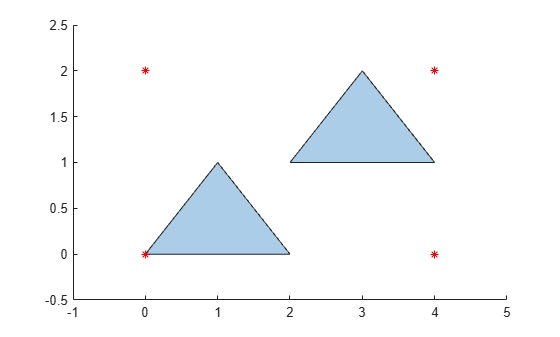boundingbox

Bounding box of polyshape

Description

example

[xlim,ylim] = boundingbox(polyin) returns the x and y bounds of the smallest rectangle enclosing a polyshape. xlim and ylim are two-element row vectors whose first elements correspond to the lower x and y bounds, and whose second elements correspond to the upper x and y bounds.

When polyin is an array of polyshape objects, xlim and ylim describe the bounding box enclosing all polyshape elements of polyin.

[xlim,ylim] = boundingbox(polyin,I) returns the bounding box limits of the Ith boundary of polyin.

This syntax is only supported when polyin is a scalar polyshape object.

Examples

collapse all

Create a polygon containing two solid regions, and compute the lower and upper x and y bounds that enclose it.

x1 = [0 1 2];
y1 = [0 1 0];
x2 = [2 3 4];
y2 = [1 2 1];
polyin = polyshape({x1,x2},{y1,y2});
[xlim,ylim] = boundingbox(polyin);
plot(polyin)
hold on
plot(xlim,ylim,'r*',xlim,fliplr(ylim),'r*')Input Arguments

collapse all

Input polyshape, specified as a scalar, vector, matrix, or multidimensional array.

Data Types: polyshape

Boundary index, specified as a scalar integer or vector of integers. Each element of I corresponds to a single boundary of the input polyshape.

When I is a vector of indices, boundingbox returns the limits enclosing all boundaries indexed by the elements of I.

Data Types: double | single | int8 | int16 | int32 | int64 | uint8 | uint16 | uint32 | uint64

Output Arguments

collapse all

x limits bounding a polyshape, returned as a two-element row vector. The first element of xlim is the lower x bound, and the second element is the upper x bound.

Data Types: double

y limits bounding a polyshape, returned as a two-element row vector. The first element of ylim is the lower y bound, and the second element is the upper y bound.

Data Types: double